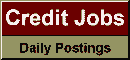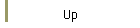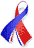DefaultRisk.com the web's biggest credit risk modeling resource.doi> search: A or B Export citation to:- HTML- Text (plain)- BibTeX- RIS- ReDIF

Regression Models for Ordinal Data

by Peter McCullagh of the University of Chicago

1980

Summary: A General class of regression models for ordinal data is developed and discussed. These models utilize the ordinal nature of the data by describing various modes of stochastic ordering and this eliminates the need for assigning scores or otherwise assuming cardinality instead of ordinality. Two models in particular, the proportional odds and the proportional hazards models are likely to be most useful in practice because of the simplicity of their interpretation. These linear models are shown to be multivariate extensions of generalized linear models. Extensions to non-linear models are discussed and it is shown that even here the method of iteratively reweighted least squares converges to the maximum likelihood estimate, a property which greatly simplifies the necessary computation. Applications are discussed with the aid of examples.

Keywords: Complementary log-log transform, generalized empirical logit transform, link function, location parameter, log-linear model, logit linear model, multivariate generalized linear model, ordered categories, proportional hazards, proportional odds, scale parameter, scores, survivor function.

Published in: Journal of the Royal Statistical Society, Series B (Methodology), Vol. 42, No. 2, (1980), pp. 109-142.

Books Referenced in this paper:  (what is this?)